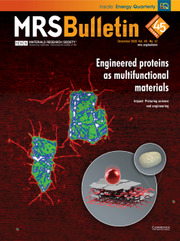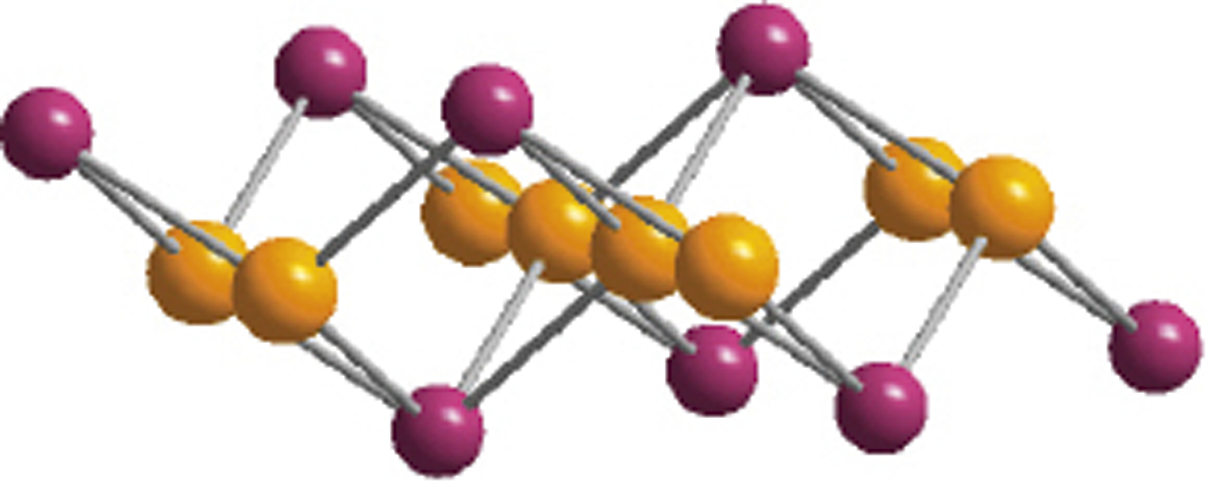Home
Hostname: page-component-544b6db54f-4nk8m Total loading time: 0.222 Render date: 2021-10-23T20:33:12.820Z Has data issue: true Feature Flags: { "shouldUseShareProductTool": true, "shouldUseHypothesis": true, "isUnsiloEnabled": true, "metricsAbstractViews": false, "figures": true, "newCiteModal": false, "newCitedByModal": true, "newEcommerce": true, "newUsageEvents": true }MRS Bulletin

# Emergent high-temperature superconductivity at interfaces

Published online by Cambridge University Press:  07 May 2020

## AbstractLow-dimensional superconductors have been at the forefront of physics research due to their rich physical properties such as high-temperature (Tc) superconductivity. In this article, we review the field of emergent high-Tc superconductivity at interfaces of heterostructures, focusing on the experimental advances and its physical mechanism. Charge transfer between constituent materials leads to two-dimensional carrier confinement that facilitates occurrence of superconductivity at the interface. We discuss the similarities between bulk high-Tc superconductors and interface systems, as well as implications from a survey of interface superconductors. We expect that the hybrid heterostructures and the ability to manipulate them on an atomic scale could be an enormously fertile ground to explore superconductivity with higher critical temperature Tc.

Type
Emergent Quantum Materials
Information
MRS Bulletin , May 2020 , pp. 366 - 372

## Access options

Get access to the full version of this content by using one of the access options below. (Log in options will check for institutional or personal access. Content may require purchase if you do not have access.)

## References

Reyren, N., Thiel, S., Caviglia, A.D., Kourkoutis, L.F., Hammerl, G., Richter, C., Schneider, C.W., Kopp, T., Rüetschi, A.S., Jaccard, D., Gabay, M., Muller, D.A., Triscone, J.M., Mannhart, J., Science 317, 1196 (2007).10.1126/science.1146006CrossRefGoogle Scholar
Gozar, A., Logvenov, G., Kourkoutis, L.F., Bollinger, A.T., Giannuzzi, L.A., Muller, D.A., Božović, I., Nature 455, 782 (2008).10.1038/nature07293CrossRefGoogle Scholar
Wang, Q.Y., Li, Z., Zhang, W.H., Zhang, Z.C., Zhang, J.S., Li, W., Ding, H., Ou, Y.B., Deng, P., Chang, K., Wen, J., Song, C.L., He, K., Jia, J.F., Ji, S.H., Wang, Y.Y., Wang, L.L., Chen, X., Ma, X.C., Xue, Q.K., Chin. Phys. Lett. 29, 037402 (2012).Google Scholar
Richter, C., Boschker, H., Dietsche, W., Fillis-Tsirakis, E., Jany, R., Loder, F., Kourkoutis, L.F., Muller, D.A., Kirtley, J.R., Schneider, C.W., Mannhart, J., Nature 502, 528 (2013).10.1038/nature12494CrossRefGoogle Scholar
Keimer, B., Kivelson, S., Norman, M., Uchida, S., Zaanen, J., Nature 518, 179 (2015).10.1038/nature14165CrossRefGoogle Scholar
Saito, Y., Nojima, T., Iwasa, Y., Nat. Rev. Mater. 2, 16094 (2017).10.1038/natrevmats.2016.94CrossRefGoogle Scholar
He, Q.L., Liu, H.C., He, M.Q., Lai, Y.H., He, H.T., Wang, G., Law, K.T., Lortz, R., Wang, J.N., Sou, I.K., Nat. Commun. 5, 4247 (2014).10.1038/ncomms5247CrossRefGoogle Scholar
Zhang, Y.M., Fan, J.Q., Wang, W.L., Zhang, D., Wang, L.L., Li, W., He, K., Song, C.L., Ma, X.C., Xue, Q.K., Phys. Rev. B 98, 220508 (2018).10.1103/PhysRevB.98.220508CrossRefGoogle Scholar
Bednorz, J.G., Müller, K.A., Z. Phys. Condens. Matter B 64, 189 (1986).10.1007/BF01303701CrossRefGoogle Scholar
Kamihara, Y., Watanabe, T., Harino, M., Hosono, H., J. Am. Chem. Soc. 130, 3296 (2008).10.1021/ja800073mCrossRefGoogle Scholar
Ginzburg, V.L., Phys. Lett. 13, 101 (1964).10.1016/0031-9163(64)90672-9CrossRefGoogle Scholar
Caviglia, A.D., Gariglio, S., Reyren, N., Jaccard, D., Schneider, T., Gabay, M., Thiel, S., Hammerl, G., Mannhart, J., Triscone, J.M., Nature 456, 624 (2008).CrossRefGoogle Scholar
Smadici, S., Lee, J.C., Wang, S., Abbamonte, P., Logvenov, G., Gozar, A., Cavellin, C.D., Božović, I., Phys. Rev. Lett. 102, 107004 (2009).10.1103/PhysRevLett.102.107004CrossRefGoogle Scholar
Wu, J., Pelleg, O., Logvenov, G., Bollinger, A.T., Sun, Y.J., Boebinger, G.S., Vanević, M., Radović, Z., Božović, I., Nat. Mater. 12, 877 (2013).10.1038/nmat3719CrossRefGoogle Scholar
Dingle, R., Störmer, H.L., Gossard, A.C., Wiegmann, W., Appl. Phys. Lett. 33, 665 (1978).CrossRefGoogle Scholar
Guo, Y., Zhang, Y.F., Bao, X.Y., Han, T.Z., Tang, Z., Zhang, L.X., Zhu, W.G., Wang, E.G., Niu, Q., Qiu, Z.Q., Jia, J.F., Zhao, Z.X., Xue, Q.K., Science 306, 1915 (2004).10.1126/science.1105130CrossRefGoogle Scholar
Qin, S., Kim, J., Niu, Q., Shih, C.K., Science 324, 1314 (2009).10.1126/science.1170775CrossRefGoogle Scholar
Zhang, T., Cheng, P., Li, W.J., Sun, Y.J., Wang, G., Zhu, X.G., He, K., Wang, L.L., Ma, X.C., Chen, X., Wang, Y.Y., Liu, Y., Lin, H.Q., Jia, J.F., Xue, Q.K., Nat. Phys. 6, 104 (2010).10.1038/nphys1499CrossRefGoogle Scholar
Zhang, H.M., Sun, Y., Li, W., Peng, J.P., Song, C.L., Xing, Y., Zhang, Q.H., Guan, J.Q., Li, Z., Zhao, Y.F., Ji, S.H., Wang, L.L., He, K., Chen, X., Gu, L., Ling, L.S., Tian, M.L., Li, L., Xie, X.C., Liu, J.P., Yang, H., Xue, Q.K., Wang, J., Ma, X.C., Phys. Rev. Lett. 114, 107003 (2015).10.1103/PhysRevLett.114.107003CrossRefGoogle Scholar
Song, C.L., Wang, Y.L., Jiang, Y.P., Li, Z., Wang, L., He, K., Chen, X., Ma, X.C., Xue, Q.K., Phys. Rev. B 84, 020503 (2011).Google Scholar
Song, C.L., Wang, Y.L., Cheng, P., Jiang, Y.P., Li, W., Zhang, T., Li, Z., He, K., Wang, L., Jia, J.F., Hung, H.H., Wu, C.J., Ma, X.C., Chen, X., Xue, Q.K., Science 332, 1410 (2011).10.1126/science.1202226CrossRefGoogle Scholar
He, S.L., He, J.F., Zhang, W.H., Zhao, L., Liu, D.F., Liu, X., Mou, D.X., Ou, Y.B., Wang, Q.Y., Li, Z., Wang, L.L., Peng, Y.Y., Liu, Y., Chen, C.Y., Yu, L., Liu, G.D., Dong, X.L., Zhang, J., Chen, C.T., Xu, Z.Y., Chen, X., Ma, X.C., Xue, Q.K., Zhou, X.J., Nat. Mater. 12, 605 (2013).CrossRefGoogle Scholar
Tan, S.Y., Zhang, Y., Xia, M., Ye, Z.R., Chen, F., Xie, X., Peng, R., Xu, D.F., Fan, Q., Xu, H.C., Jiang, J., Zhang, T., Lai, X.C., Xiang, T., Hu, J.P., Xie, N.P., Feng, D.L., Nat. Mater. 12, 634 (2013).10.1038/nmat3654CrossRefGoogle Scholar
Ge, J.F., Liu, Z.L., Liu, C.H., Gao, C.L., Qian, D., Xue, Q.K., Liu, Y., Jia, J.F., Nat. Mater. 14, 285 (2014).10.1038/nmat4153CrossRefGoogle Scholar
Mazin, I.I., Nat. Mater. 14, 755 (2015).10.1038/nmat4371CrossRefGoogle Scholar
Huang, D., Hoffman, J.E., Annu. Rev. Condens. Matter Phys. 8, 311 (2017).10.1146/annurev-conmatphys-031016-025242CrossRefGoogle Scholar
Fogel, N.Y., Buchstab, E.I., Bomze, Y.V., Yuzephovich, O.I., Mikhailov, M.Y., Sipatov, A.Y., Pashitskii, E.A., Shekhter, R.I., Jonson, M., Phys. Rev. B 73, 161306 (2006).10.1103/PhysRevB.73.161306CrossRefGoogle Scholar
Chen, Y.L., Analytis, J.G., Chu, J.H., Liu, Z.K., Mo, S.K., Qi, X.L., Zhang, H.J., Lu, D.H., Dai, X., Fang, Z., Zhang, S.C., Fisher, I.R., Hussain, Z., Shen, Z.X., Science 325, 178 (2009).CrossRefGoogle Scholar
Peng, R., Shen, X.P., Xie, X., Xu, H.C., Tan, S.Y., Xia, M., Zhang, T., Cao, H.Y., Gong, X.G., Hu, J.P., Xie, B.P., Feng, D.L., Phys. Rev. Lett. 112, 107001 (2014).10.1103/PhysRevLett.112.107001CrossRefGoogle Scholar
Berg, E., Orgad, D., Kivelson, S.A., Phys. Rev. B 78, 094509 (2008).10.1103/PhysRevB.78.094509CrossRefGoogle Scholar
Li, F.S., Zhang, Q.H., Tang, C.J., Liu, C., Shi, J.N., Nie, C.N., Zhou, G.Y., Li, Z., Zhang, W.H., Song, C.L., He, K., Ji, S.H., Zhang, S.B., Gu, L., Wang, L.L., Ma, X.C., Xue, Q.K., 2D Materials 3 , 024002 (2016).Google Scholar
Ding, H., Lv, Y.F., Zhao, K., Wang, W.L., Wang, L.L., Song, C.L., Chen, X., Ma, X.C., Xue, Q.K., Phys. Rev. Lett. 117, 067001 (2016).Google Scholar
Zhang, H.M., Zhang, D., Lu, X.W., Liu, C., Zhou, G.Y., Ma, X.C., Wang, L.L., Jiang, P., Xue, Q.K., Bao, X.H., Nat. Commun. 8, 214 (2017).CrossRefGoogle Scholar
Zhao, W.W., Li, M.D., Chang, C.Z., Jiang, J., Wu, L.J., Liu, C.X., Moodera, J.S., Zhu, Y.M., Chan, M.H.W., Sci. Adv. 4, eaa02683 (2018).Google Scholar
Miyata, Y., Nakayama, K., Sugawara, K., Sato, T., Takahashi, T., Nat. Mater. 14, 775 (2015).CrossRefGoogle Scholar
Song, C.L., Zhang, H.M., Zhong, Y., Hu, X.P., Ji, S.H., Wang, L., He, K., Ma, X.C., Xue, Q.K., Phys. Rev. Lett. 116, 157001 (2016).10.1103/PhysRevLett.116.157001CrossRefGoogle Scholar
Lei, B., Cui, J.H., Xiang, Z.J., Shang, C., Wang, N.Z., Ye, G.J., Luo, X.G., Wu, T., Sun, Z., Chen, X.H., Phys. Rev. Lett. 116, 077002 (2016).CrossRefGoogle Scholar
Burrard-Lucas, M., Free, D.G., Sedlmaier, S.J., Wright, J.D., Cassidy, S.J., Hara, Y., Corkett, A.J., Lancaster, T., Baker, P.J., Blundell, S.J., Clarke, S.J., Nat. Mater. 12, 15 (2013).10.1038/nmat3464CrossRefGoogle Scholar
Lu, X.F., Wang, N.Z., Wu, H., Wu, Y.P., Zhao, D., Zeng, X.Z., Luo, X.G., Wu, T., Bao, W., Zhang, G.H., Chen, X.H., Nat. Mater. 14, 325 (2015).10.1038/nmat4155CrossRefGoogle Scholar
Lee, D.H., Chin. Phys. B 24, 117405 (2015).CrossRefGoogle Scholar
Song, Q., Yu, T.L., Lou, X., Xie, B.P., Xu, H.C., Wen, C.H.P., Yao, Q., Zhang, S.Y., Zhu, X.T., Guo, J.D., Peng, R., Feng, D.L., Nat. Commun. 10, 758 (2019).10.1038/s41467-019-08560-zCrossRefGoogle Scholar
Lee, J.J., Schmitt, F.T., Moore, R.G., Johnston, S., Cui, Y.T., Li, W., Yi, M., Liu, Z.K., Hashimoto, M., Zhang, Y., Lu, D.H., Devereaux, T.P., Lee, D.H., Shen, Z.X., Nature 515, 245 (2014).10.1038/nature13894CrossRefGoogle Scholar
Jiang, D.Y., Hu, T., You, L.X., Li, Q.M., Li, A., Wang, H.M., Mu, G., Chen, Z.Y., Zhang, H.R., Yu, G.H., Zhu, J., Sun, Q.J., Lin, C.T., Xiao, H., Xie, X.M., Jiang, M.H., Nat. Commun. 5, 5708 (2014).10.1038/ncomms6708CrossRefGoogle Scholar
Yu, Y.J., Ma, L.G., Cai, P., Zhong, R.D., Ye, C., Shen, J., Gu, G.D., Chen, X.H., Zhang, Y.B., Nature 575, 156 (2019).10.1038/s41586-019-1718-xCrossRefGoogle Scholar
Zhong, Y., Wang, Y., Han, S., Lv, Y.F., Wang, W.L., Zhang, D., Ding, H., Zhang, Y.M., Wang, L., He, K., Zhong, R.D., Schneeloch, J.A., Gu, G.D., Song, C.L., Ma, X.C., Xue, Q.K., Sci. Bull. 61, 1239 (2016).10.1007/s11434-016-1145-4CrossRefGoogle Scholar
Bardeen, J., Cooper, L.N., Schrieffer, J.R., Phys. Rev. 106, 162 (1957).10.1103/PhysRev.106.162CrossRefGoogle Scholar
Mazin, I.I., Singh, D.J., Johannes, M.D., Du, M.H., Phys. Rev. Lett. 101, 057003 (2008).Google Scholar
Kuroki, K., Onari, S., Arita, R., Usui, H., Tanaka, Y., Kontani, H., Aoki, H., Phys. Rev. Lett. 101, 087004 (2008).10.1103/PhysRevLett.101.087004CrossRefGoogle Scholar
Fan, Q., Zhang, W.H., Liu, X., Yan, Y.J., Ren, M.Q., Peng, R., Xu, H.C., Xie, B.P., Hu, J.P., Zhang, T., Feng, D.L., Nat. Phys. 11, 946 (2015).10.1038/nphys3450CrossRefGoogle Scholar
Du, Z.Y., Yang, X., Altenfeld, D., Gu, Q.Q., Yang, H., Eremin, I., Hirschfeld, P.J., Mazin, I.I., Lin, H., Zhu, X.Y., Wen, H.H., Nat. Phys. 14, 134 (2018).CrossRefGoogle Scholar
Jiang, K., Wu, X.X., Hu, J.P., Wang, Z.Q., Phys. Rev. Lett. 121, 227002 (2018).10.1103/PhysRevLett.121.227002CrossRefGoogle Scholar
Wang, Z.F., Zhang, H.M., Liu, D.F., Liu, C., Tang, C.J., Song, C.L., Zhong, Y., Peng, J.P., Li, F.S., Nie, C.N., Wang, L.L., Zhou, X.J., Ma, X.C., Xue, Q.K., Liu, F., Nat. Mater. 15, 968 (2016).10.1038/nmat4686CrossRefGoogle Scholar
Lu, J.M., Zheliuk, O., Leermarkers, I., Yuan, N.F.Q., Zeitler, U., Law, K.T., Ye, J.T., Science 350, 1353 (2015).10.1126/science.aab2277CrossRefGoogle Scholar

# Send article to Kindle

Note you can select to send to either the @free.kindle.com or @kindle.com variations. ‘@free.kindle.com’ emails are free but can only be sent to your device when it is connected to wi-fi. ‘@kindle.com’ emails can be delivered even when you are not connected to wi-fi, but note that service fees apply.

Find out more about the Kindle Personal Document Service.

Emergent high-temperature superconductivity at interfaces
Available formats
×

# Send article to Dropbox

To send this article to your Dropbox account, please select one or more formats and confirm that you agree to abide by our usage policies. If this is the first time you use this feature, you will be asked to authorise Cambridge Core to connect with your <service> account. Find out more about sending content to Dropbox.

Emergent high-temperature superconductivity at interfaces
Available formats
×

# Send article to Google Drive

To send this article to your Google Drive account, please select one or more formats and confirm that you agree to abide by our usage policies. If this is the first time you use this feature, you will be asked to authorise Cambridge Core to connect with your <service> account. Find out more about sending content to Google Drive.

Emergent high-temperature superconductivity at interfaces
Available formats
×
×